# Basic commutator

An object inductively constructed from the elements of a given set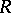and from brackets, in the following manner. The elements ofare considered by definition to be basic commutators of length 1, and they are given an arbitrary total order. The basic commutators of length, where whereis an integer, are defined and ordered as follows. Ifare basic commutators of lengths smaller than, then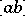is considered to be a basic commutator of lengthif and only if the following conditions are met: 1)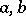are basic commutators of lengthsand, respectively, and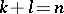; 2)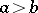; and 3) if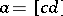, then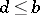. The basic commutators of length not exceedingthus obtained are arbitrarily ordered, subject to the condition that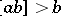, while preserving the order of the basic commutators of lengths less than. Any set of basic commutators constructed in this way is a base of the free Lie algebra withas set of free generators .